# NCERT Exemplar Class 12 Chemistry Solutions for Chapter 10 - Haloalkanes and Haloarenes

The NCERT Class 12 Chemistry Exemplar for Chapter 10 Haloalkanes and Haloarenes helps you get complete knowledge about haloarenes and haloalkanes. After going through the NCERT Class 12 Chemistry Exemplar for Chapter 10, students will get a clear picture of the reactions involved in the preparation of haloalkanes and haloarenes and understand the various reactions that they undergo.

## Download the PDF of NCERT Exemplar for Class 12 Chemistry Chapter 10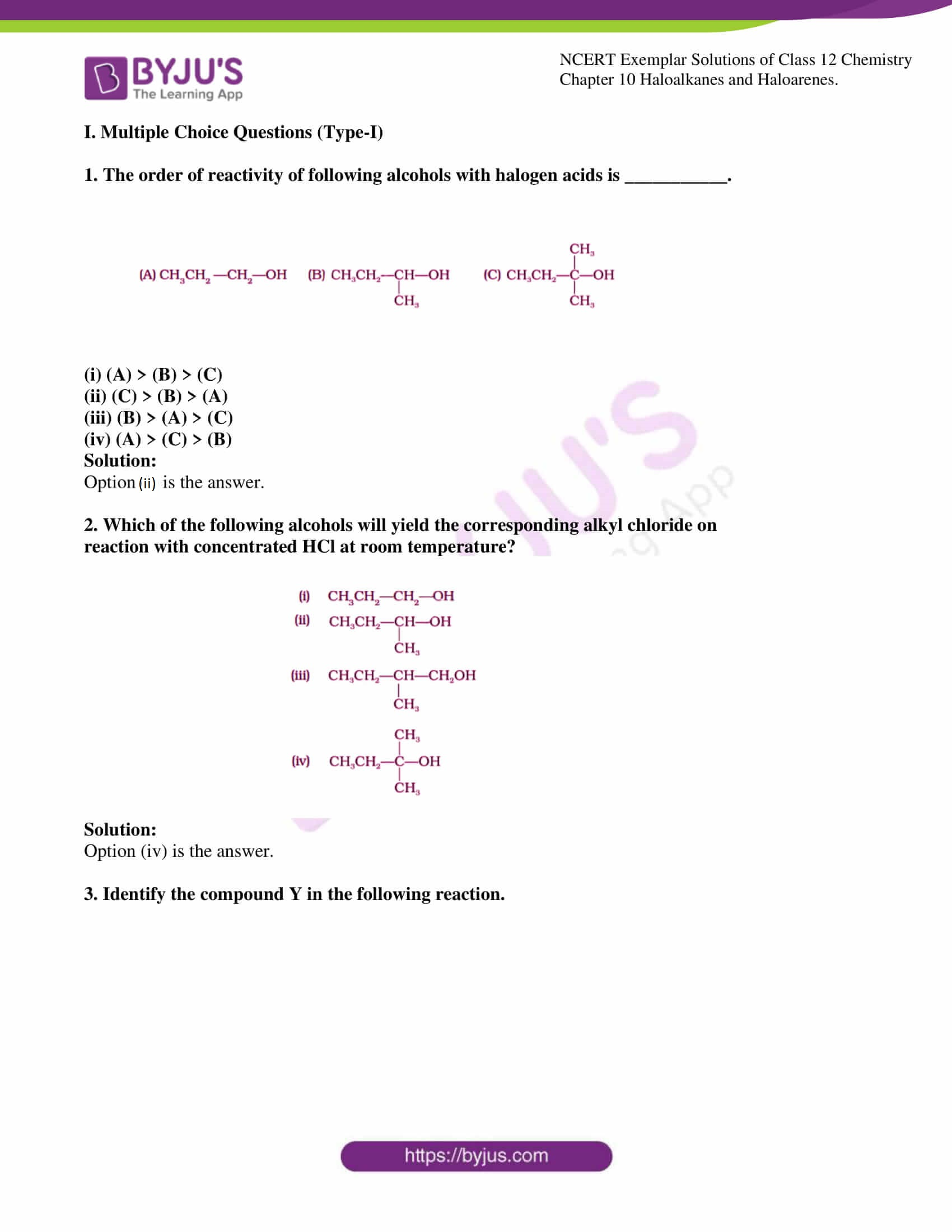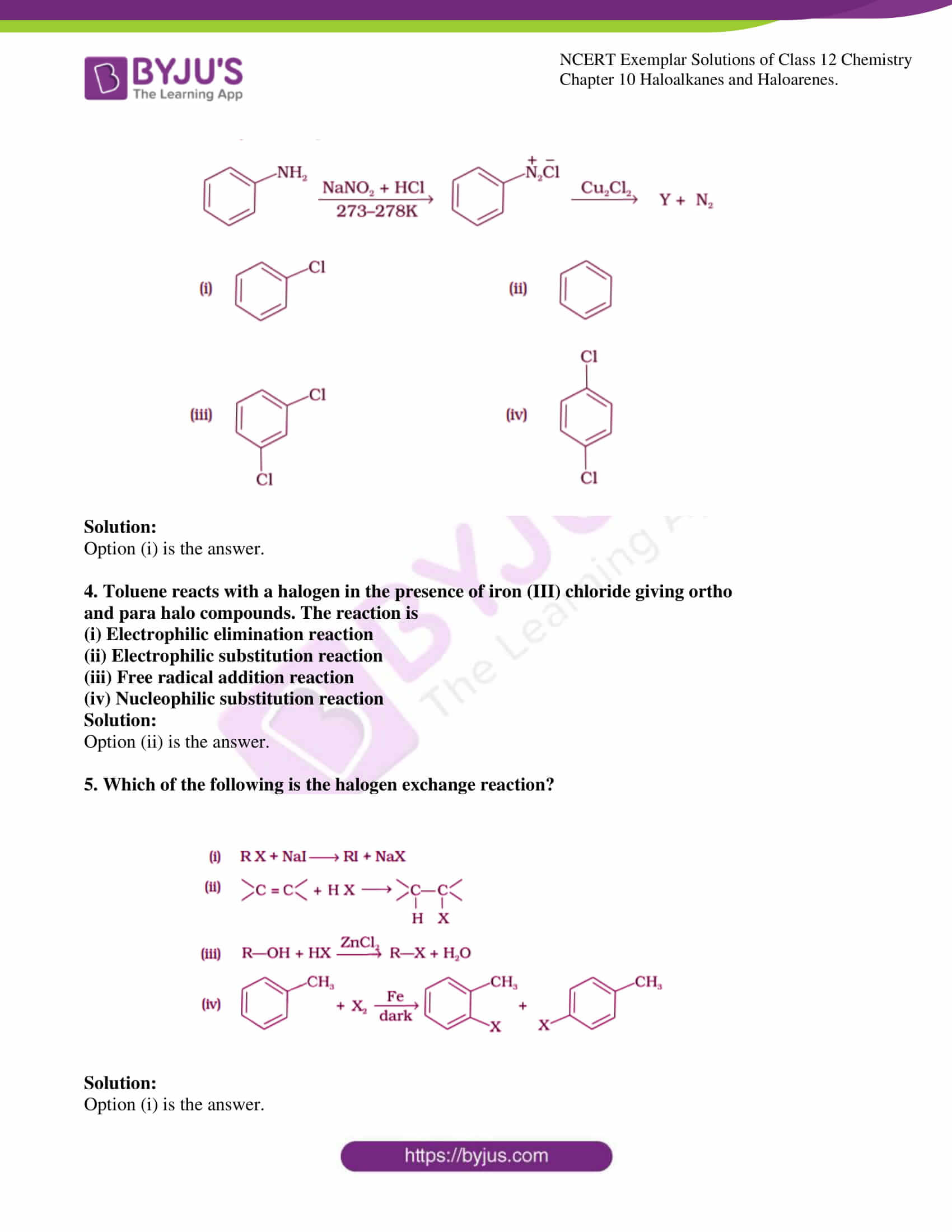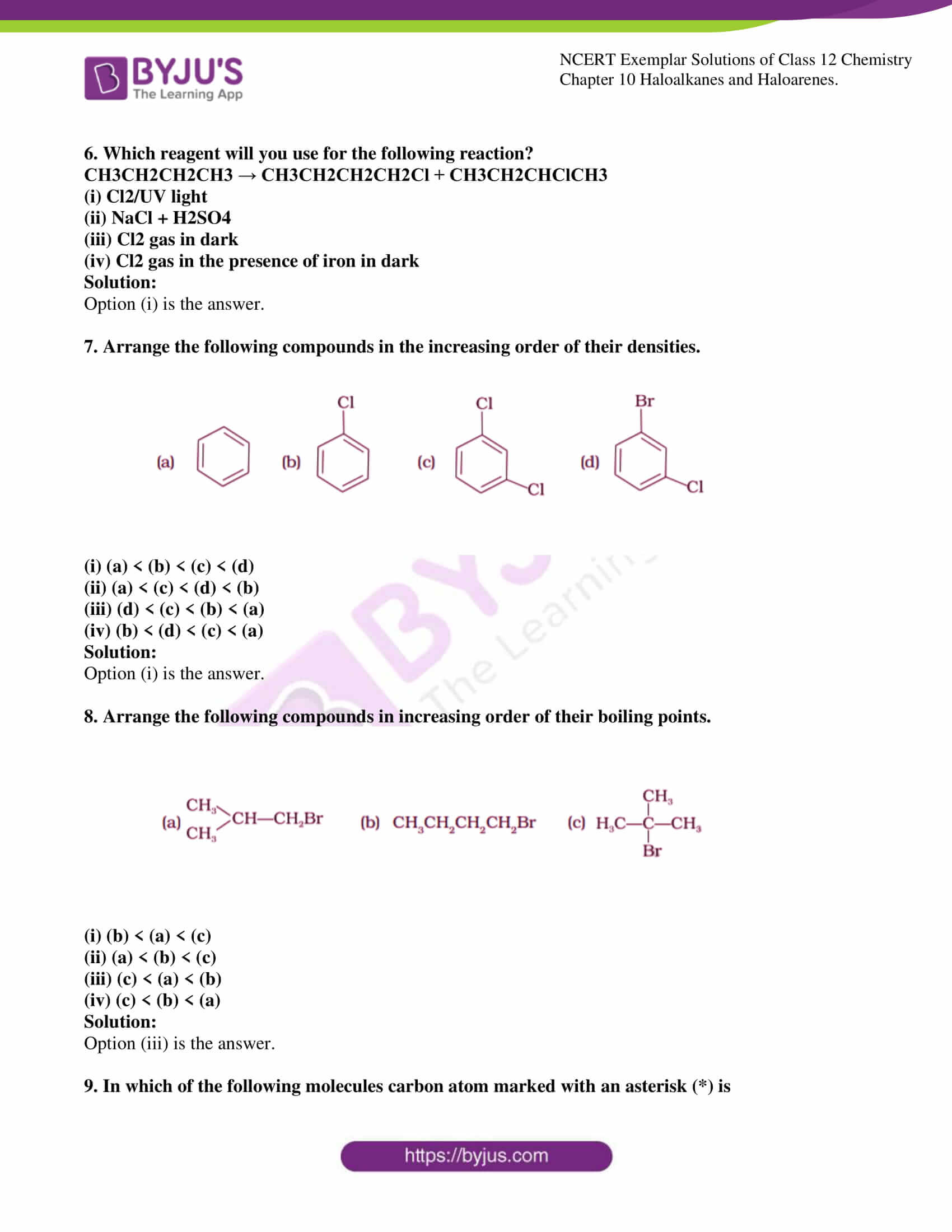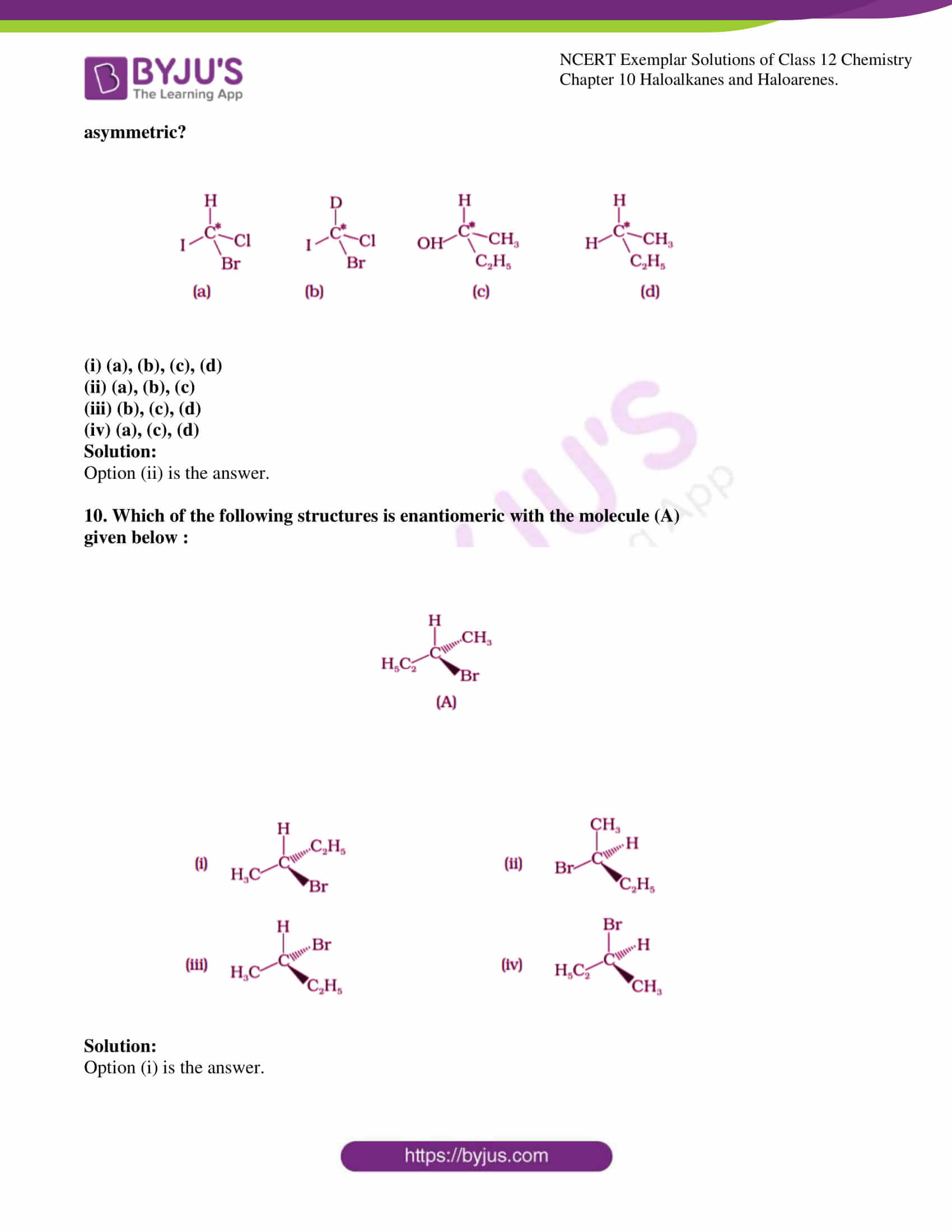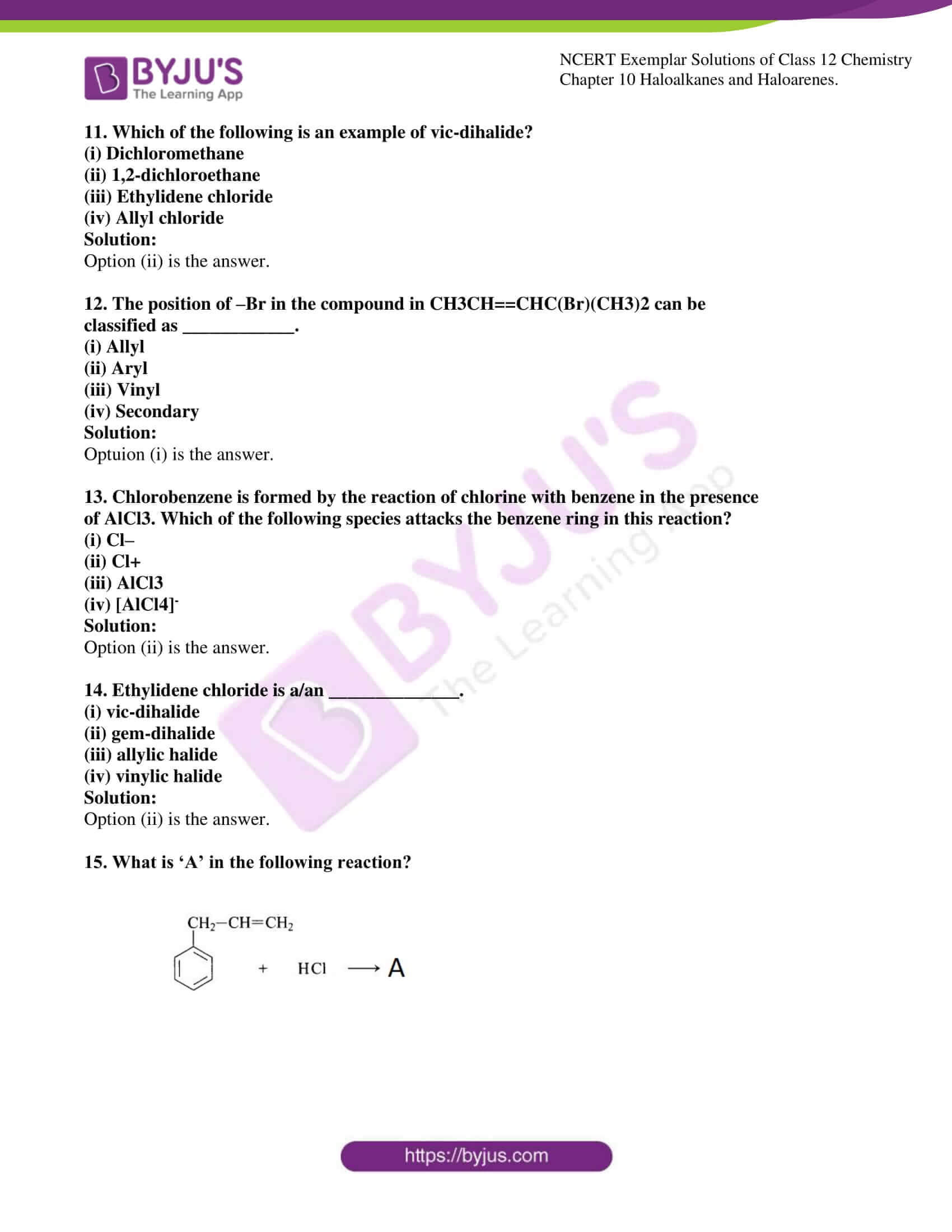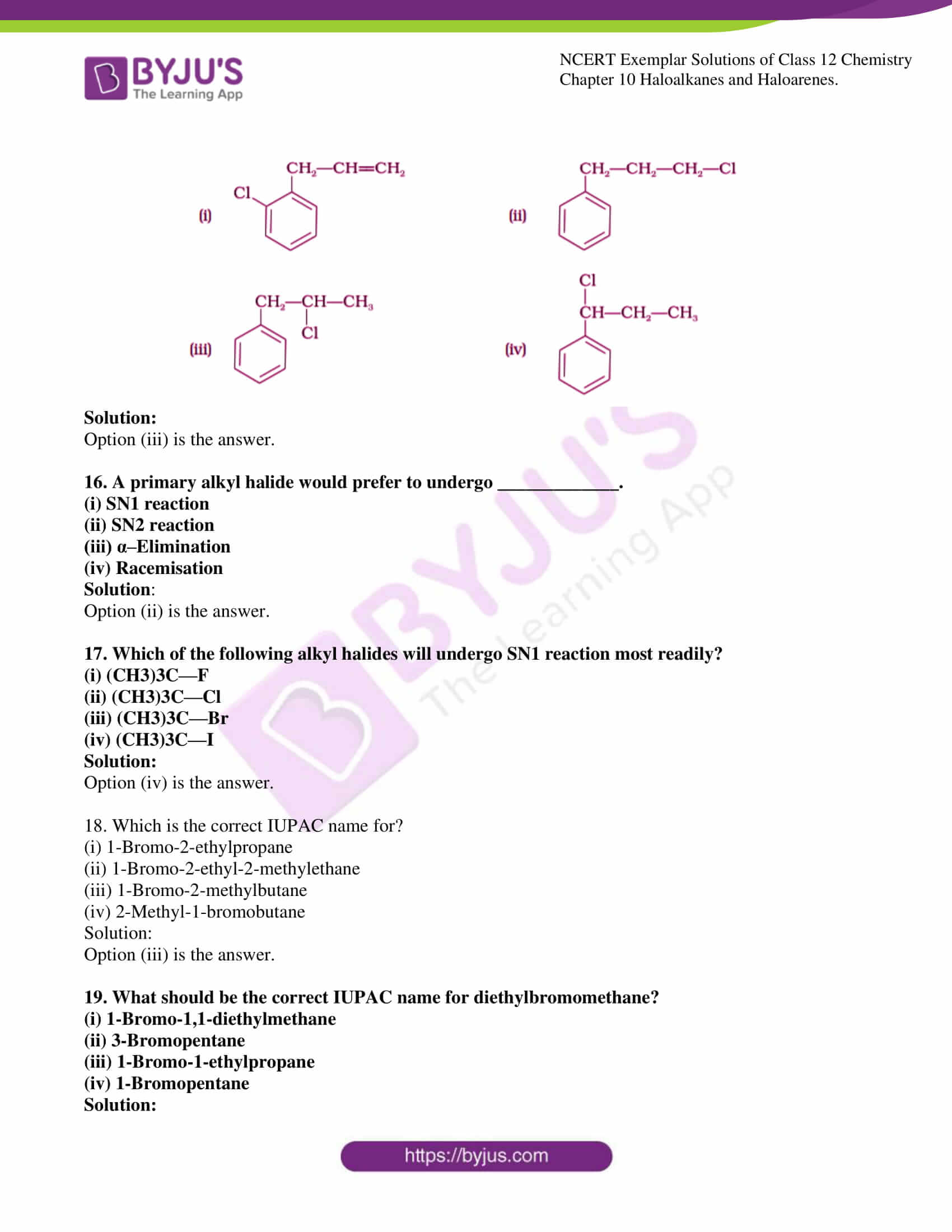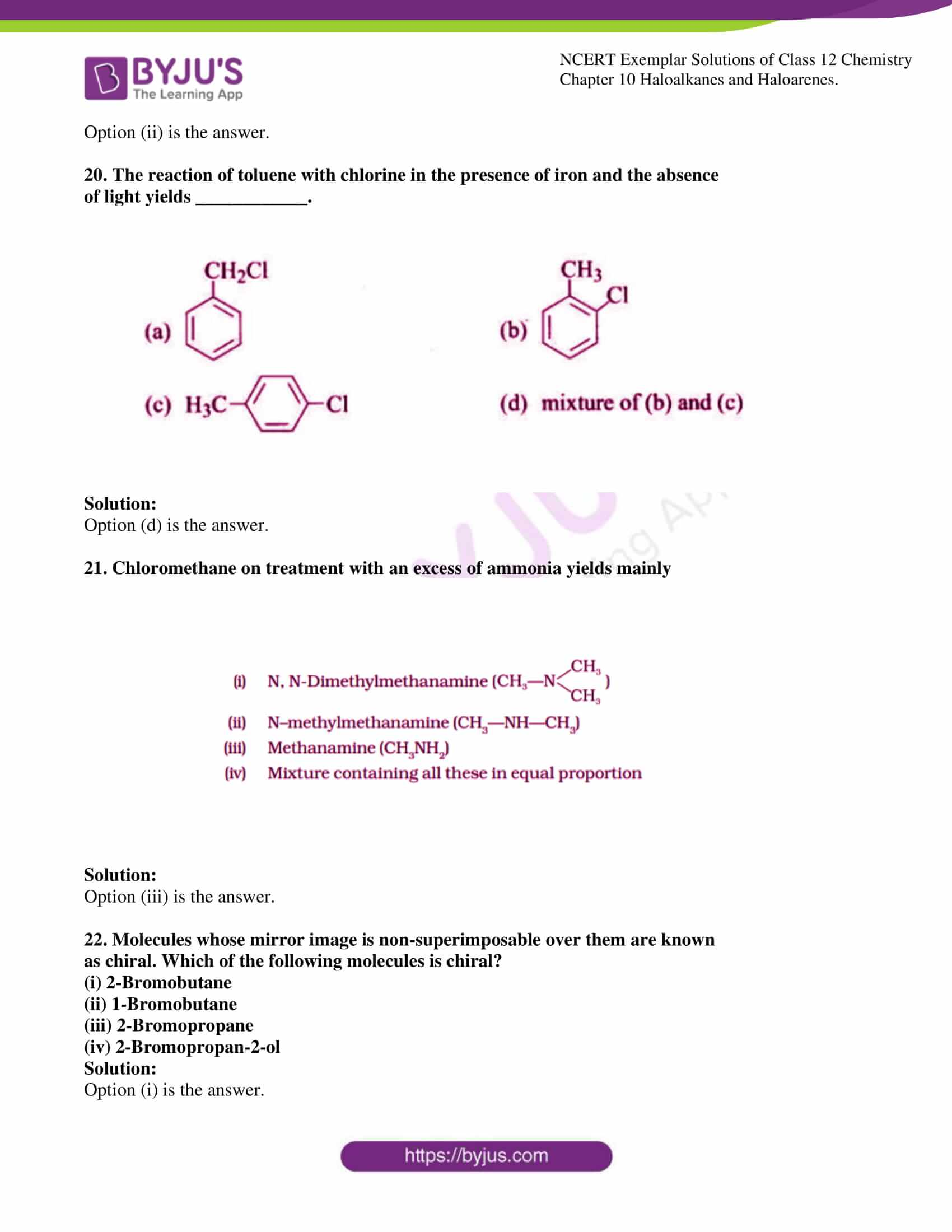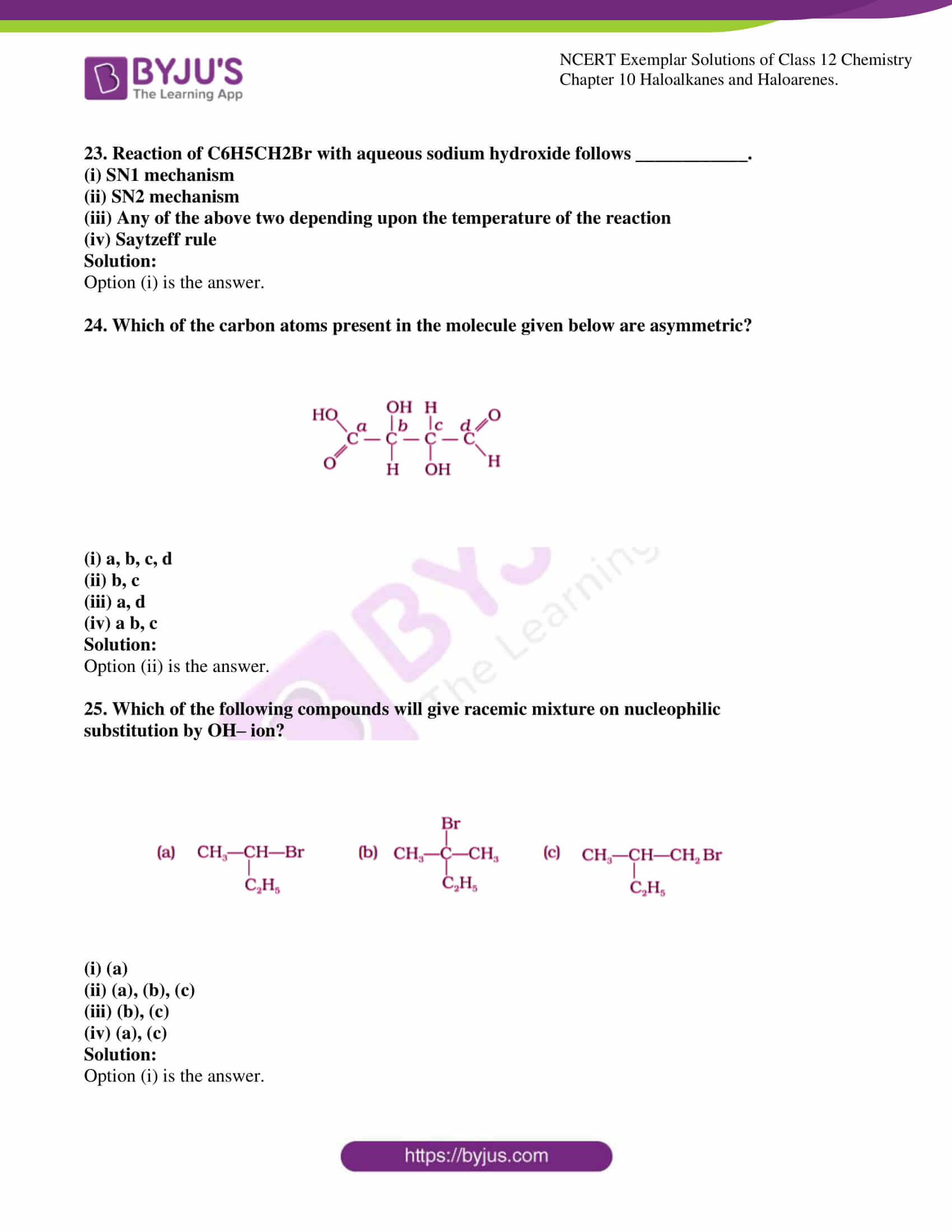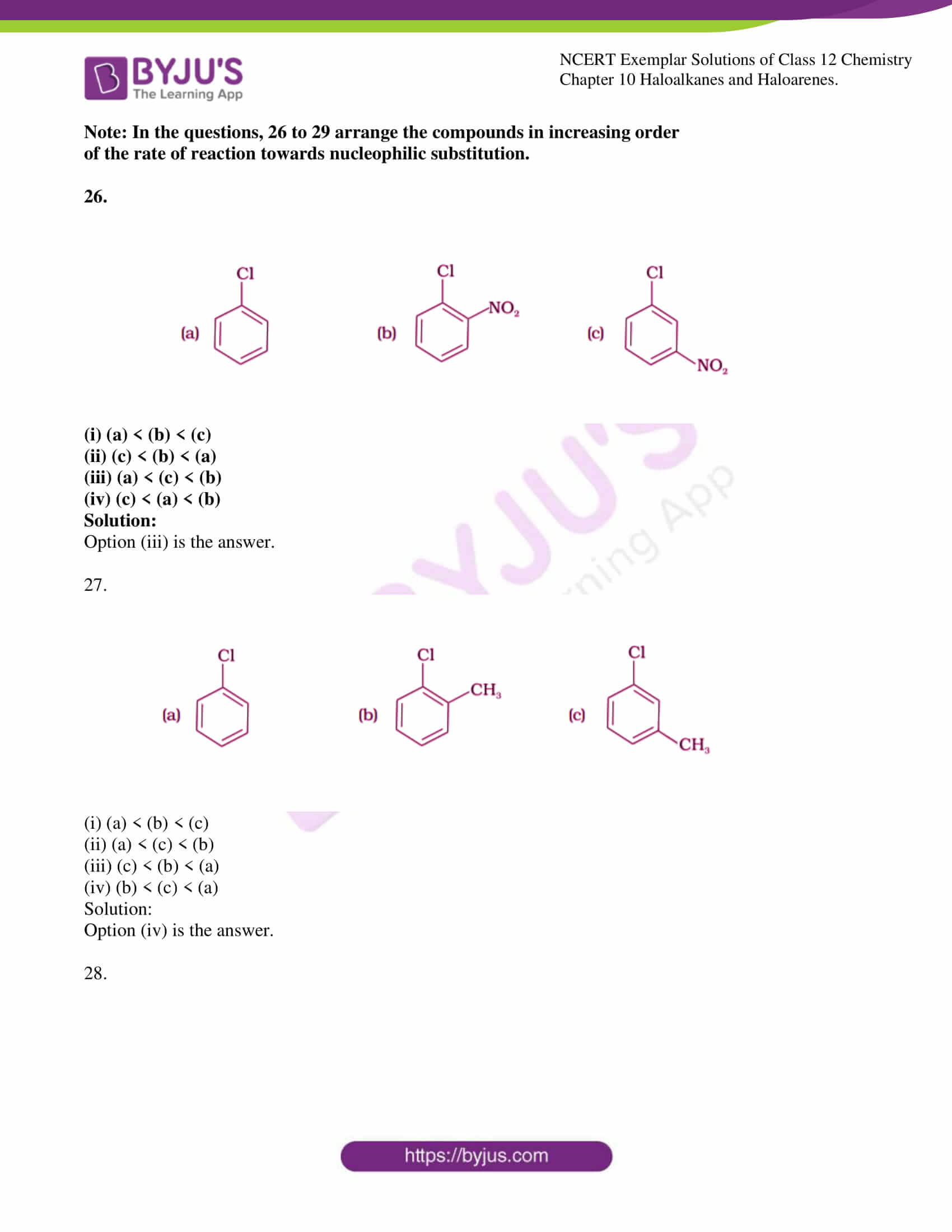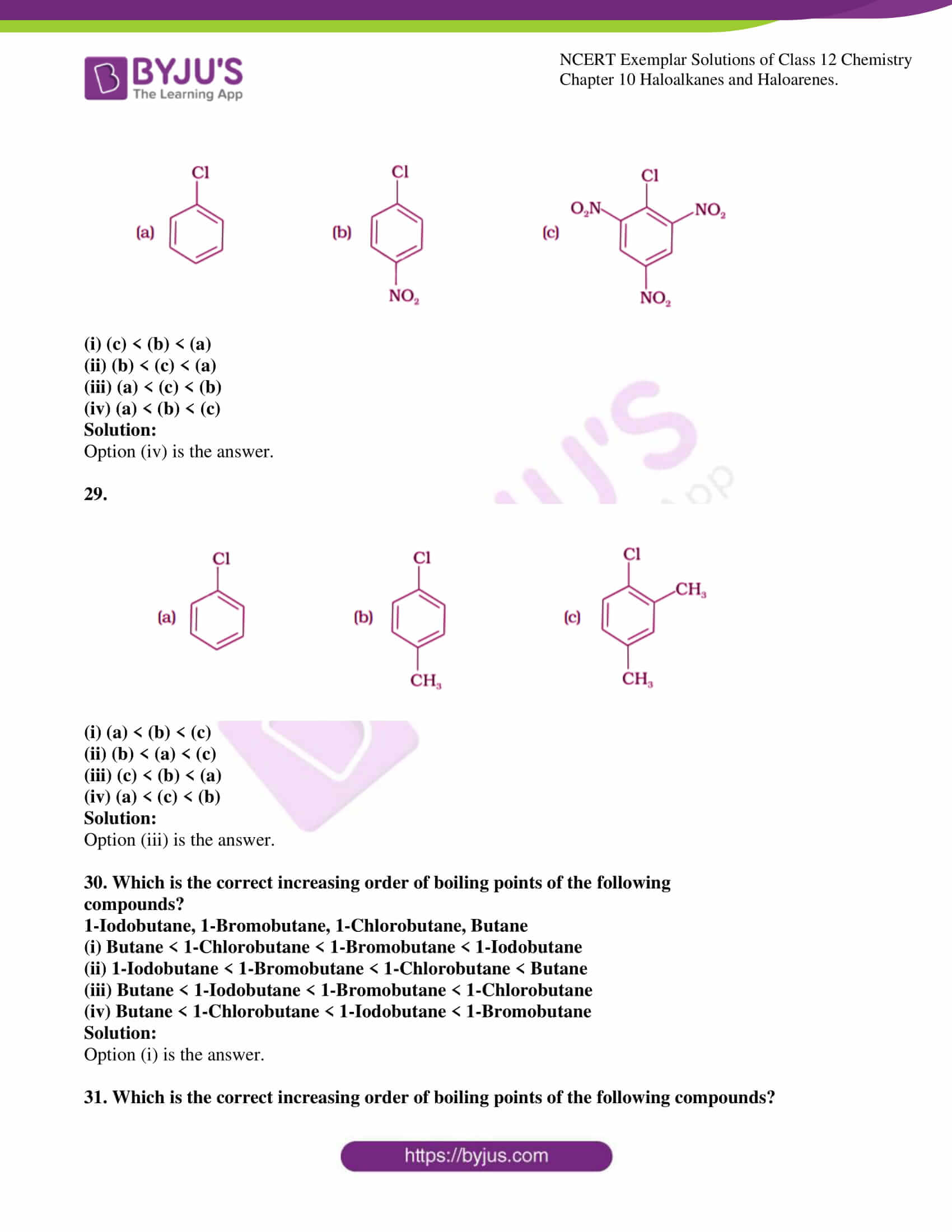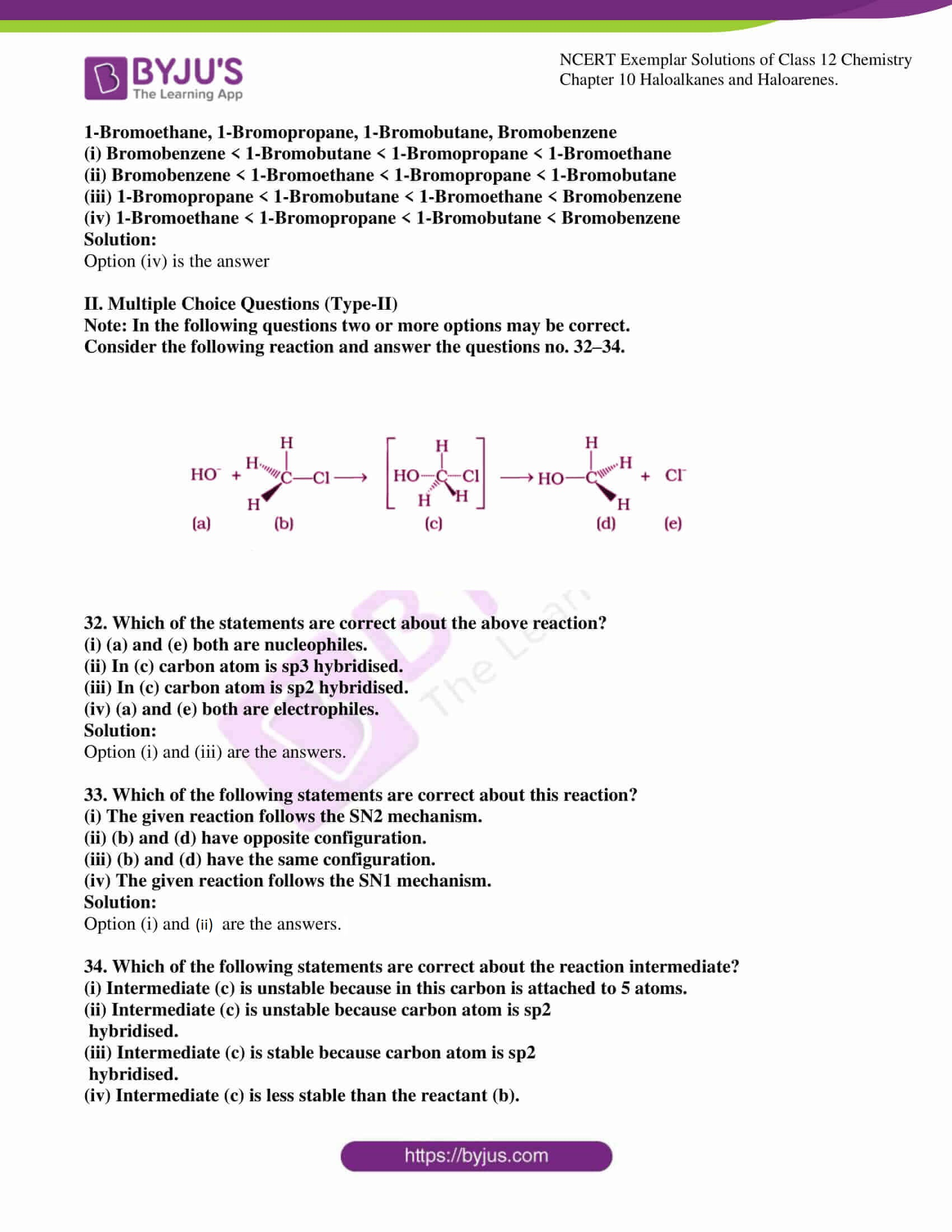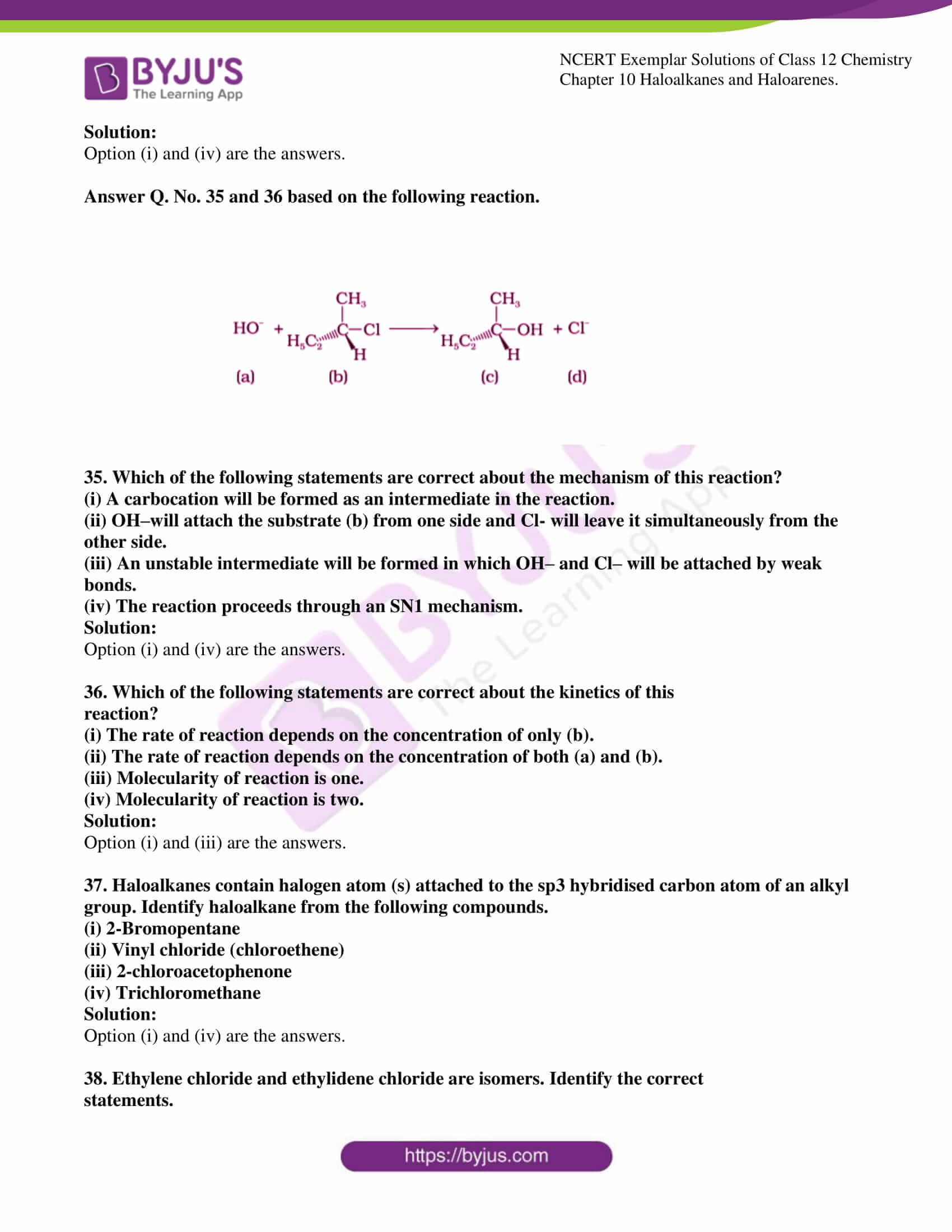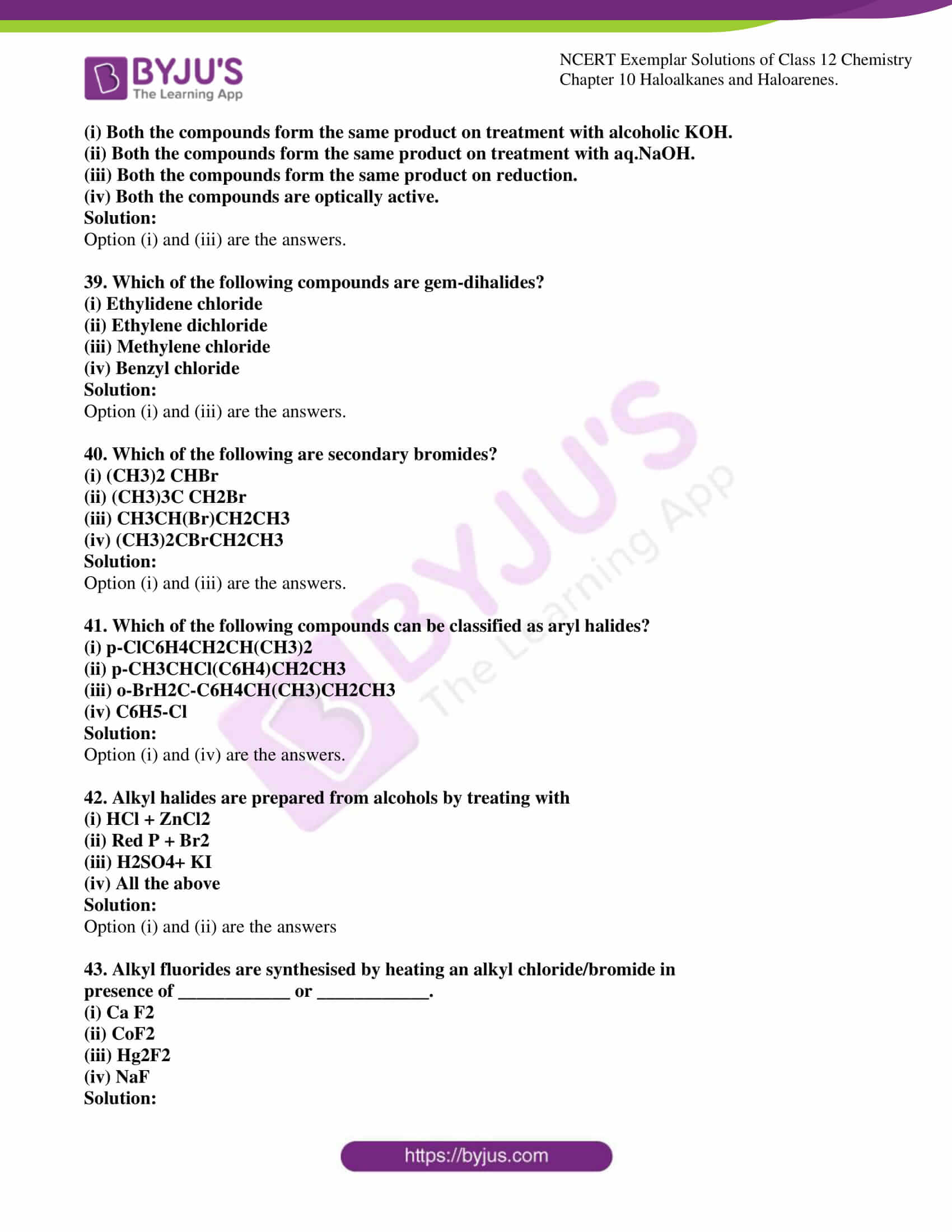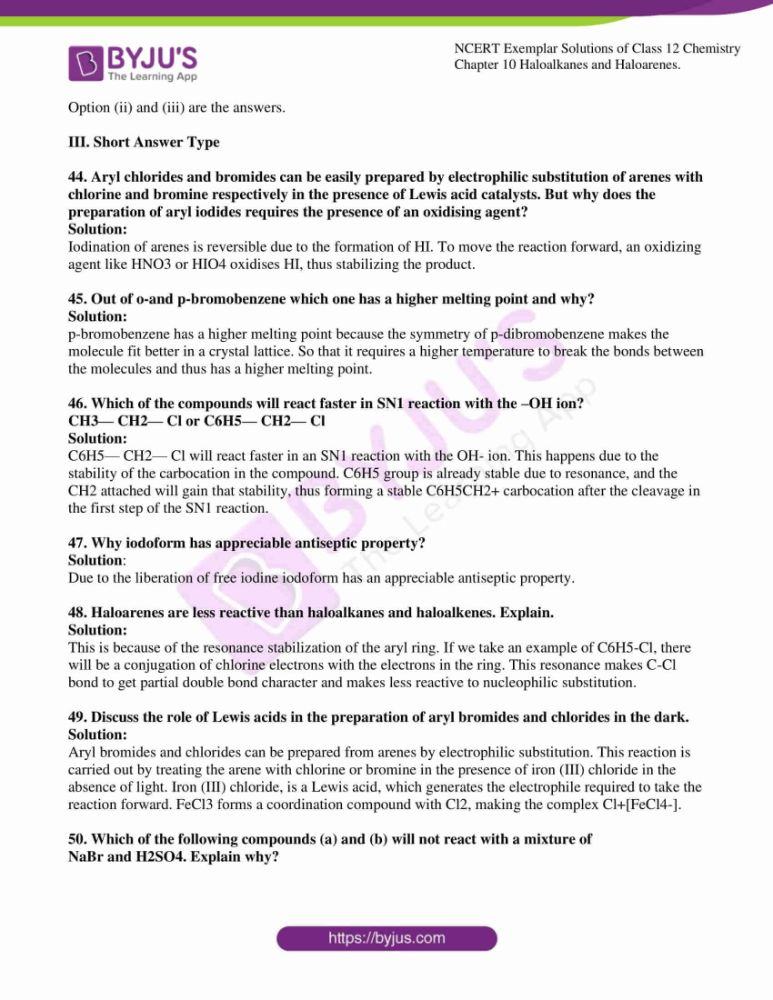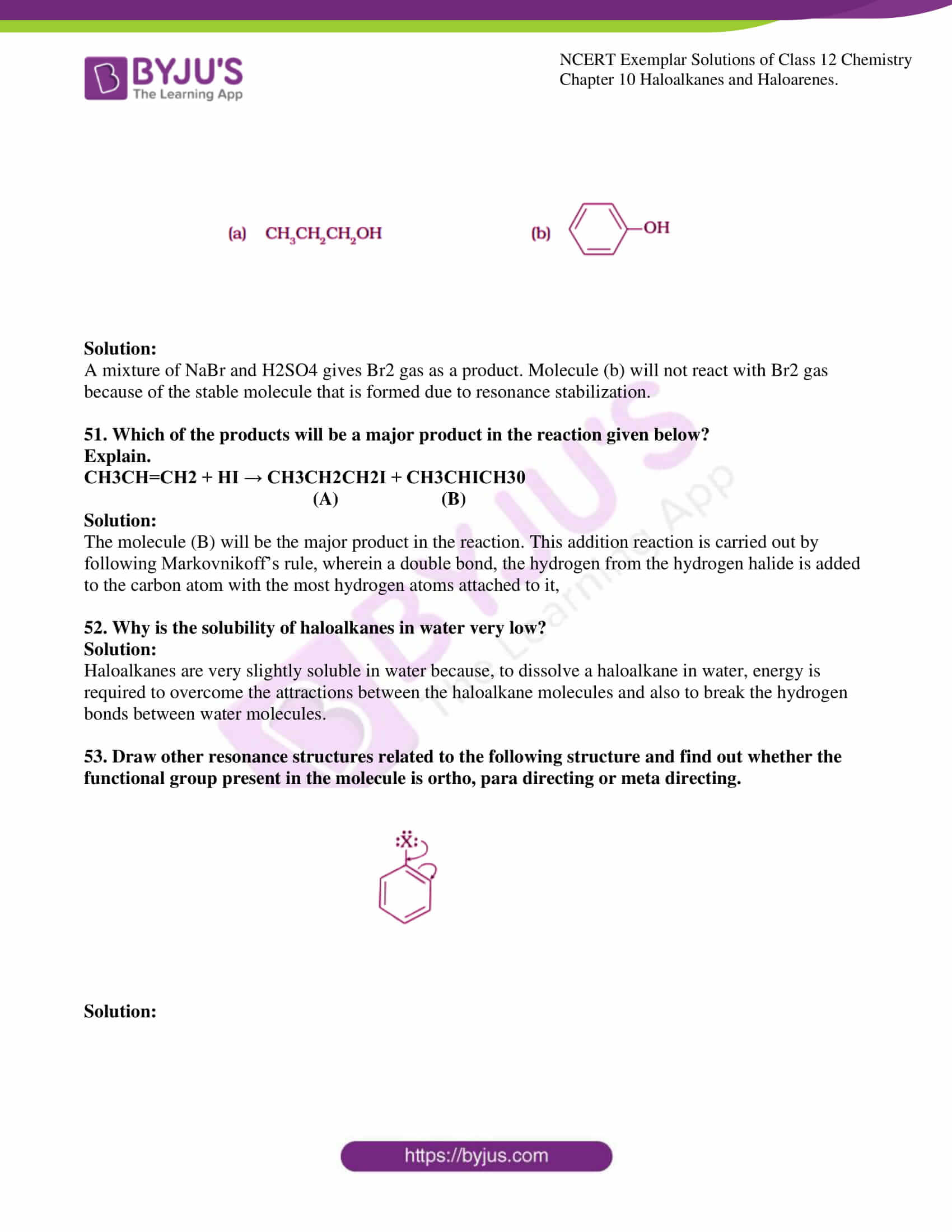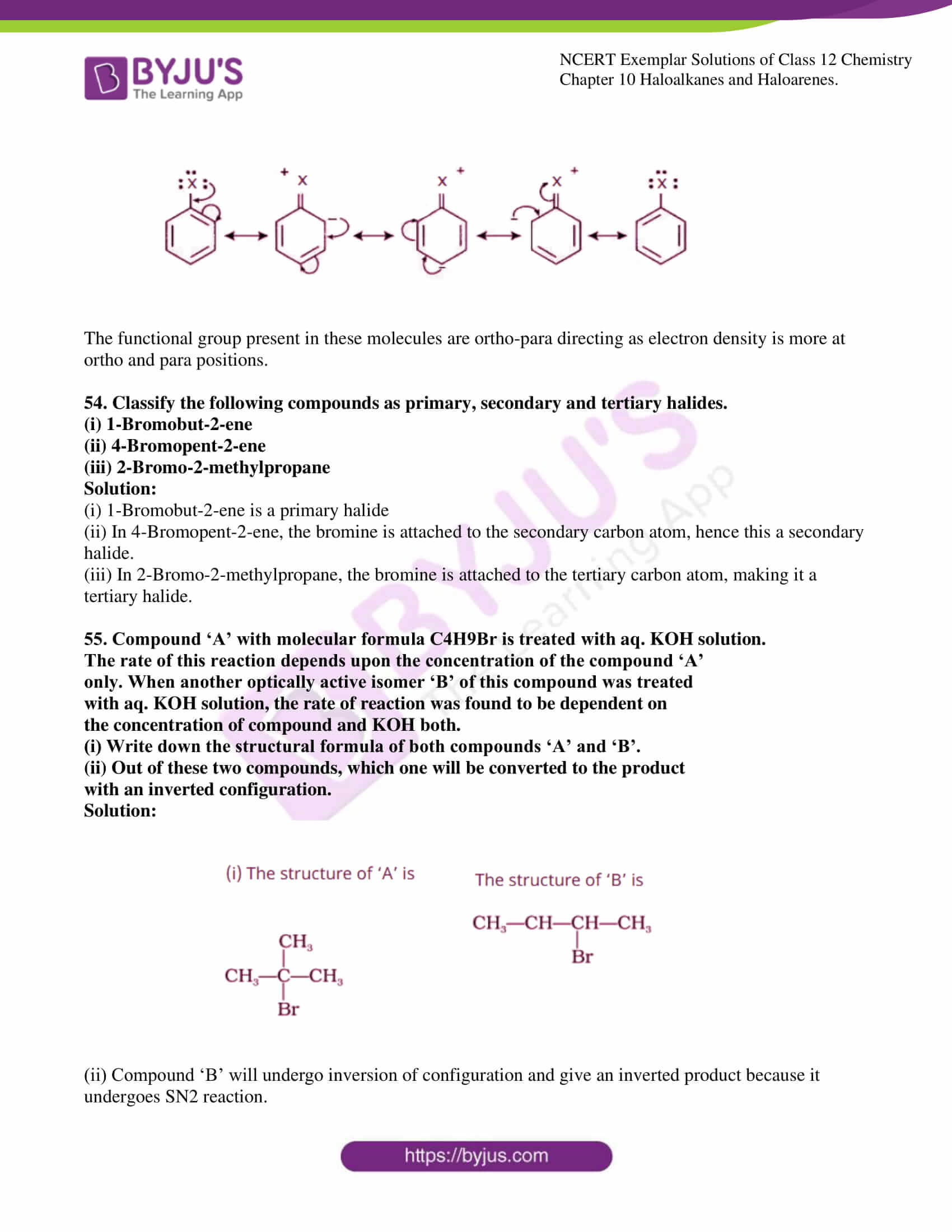### Access Solutions to the NCERT Exemplar Class 12 Chemistry Chapter 10

I. Multiple Choice Questions (Type-I)

1. The order of reactivity of following alcohols with halogen acids is ___________.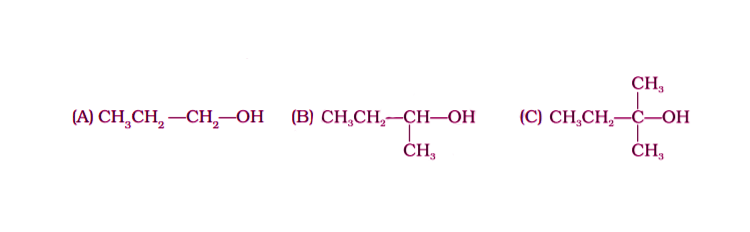(i) (A) > (B) > (C)

(ii) (C) > (B) > (A)

(iii) (B) > (A) > (C)

(iv) (A) > (C) > (B)

Solution:

2. Which of the following alcohols will yield the corresponding alkyl chloride on

reaction with concentrated HCl at room temperature?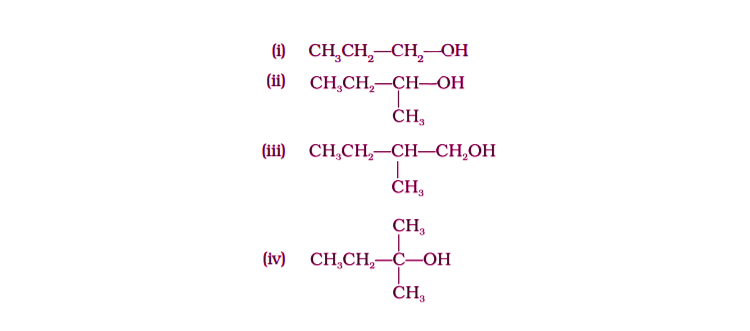Solution:

3. Identify the compound Y in the following reaction.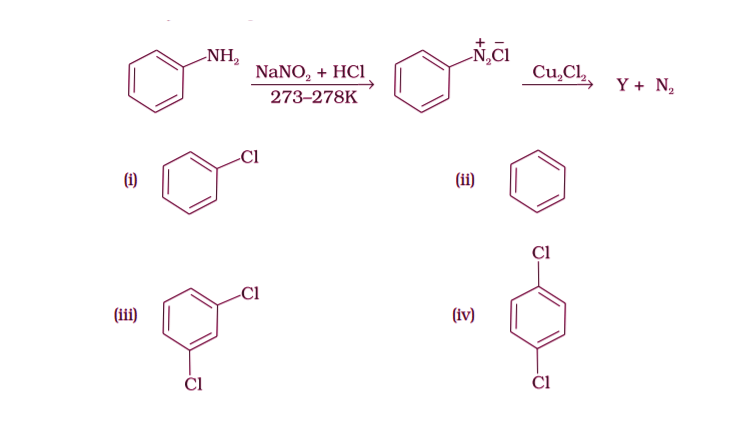Solution:

4. Toluene reacts with a halogen in the presence of iron (III) chloride giving ortho

and para halo compounds. The reaction is

(i) Electrophilic elimination reaction

(ii) Electrophilic substitution reaction

(iv) Nucleophilic substitution reaction

Solution:

5. Which of the following is the halogen exchange reaction?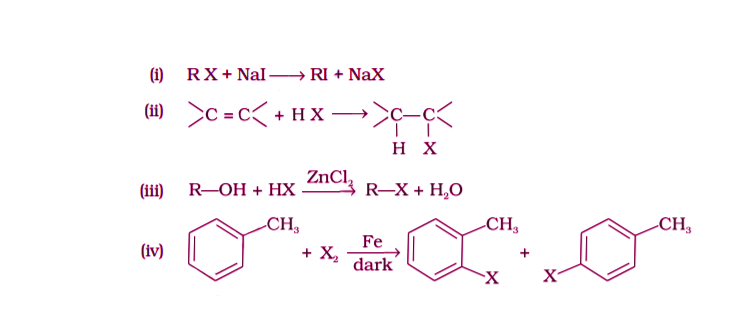Solution:

6. Which reagent will you use for the following reaction?

CH3CH2CH2CH3 → CH3CH2CH2CH2Cl + CH3CH2CHClCH3

(i) Cl2/UV light

(ii) NaCl + H2SO4

(iii) Cl2 gas in dark

(iv) Cl2 gas in the presence of iron in dark

Solution:

7. Arrange the following compounds in the increasing order of their densities.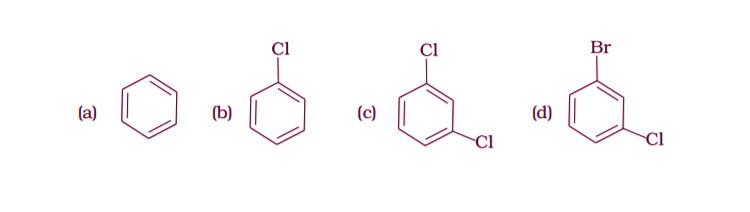(i) (a) < (b) < (c) < (d)

(ii) (a) < (c) < (d) < (b)

(iii) (d) < (c) < (b) < (a)

(iv) (b) < (d) < (c) < (a)

Solution:

8. Arrange the following compounds in increasing order of their boiling points.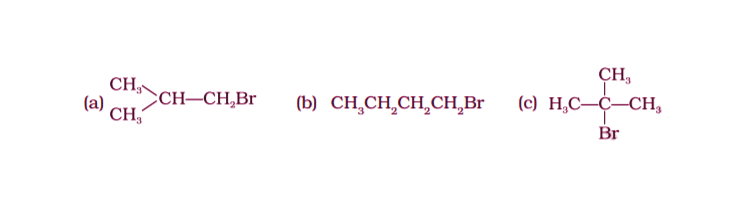(i) (b) < (a) < (c)

(ii) (a) < (b) < (c)

(iii) (c) < (a) < (b)

(iv) (c) < (b) < (a)

Solution:

9. In which of the following molecules carbon atom marked with an asterisk (*) is

asymmetric?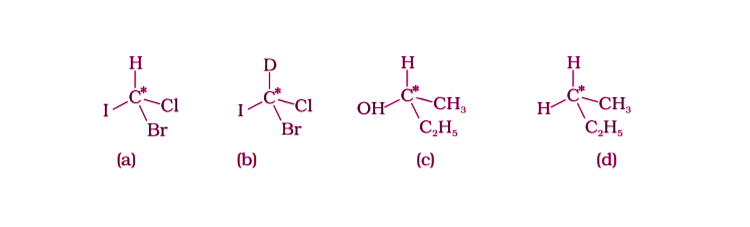(i) (a), (b), (c), (d)

(ii) (a), (b), (c)

(iii) (b), (c), (d)

(iv) (a), (c), (d)

Solution:

10. Which of the following structures is enantiomeric with the molecule (A)

given below :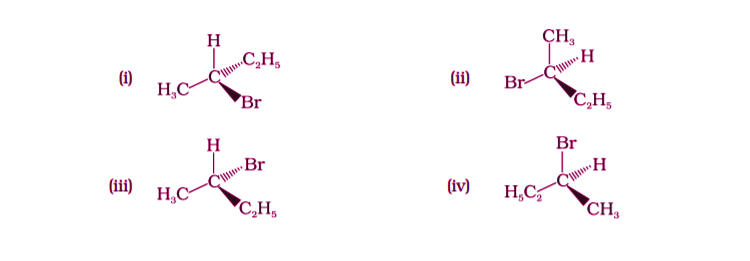Solution:

11. Which of the following is an example of vic-dihalide?

(i) Dichloromethane

(ii) 1,2-dichloroethane

(iii) Ethylidene chloride

(iv) Allyl chloride

Solution:

12. The position of –Br in the compound in CH3CH==CHC(Br)(CH3)2 can be

classified as ____________.

(i) Allyl

(ii) Aryl

(iii) Vinyl

(iv) Secondary

Solution:

13. Chlorobenzene is formed by the reaction of chlorine with benzene in the presence

of AlCl3. Which of the following species attacks the benzene ring in this reaction?

(i) Cl–

(ii) Cl+

(iii) AlCl3

(iv) [AlCl4]

Solution:

14. Ethylidene chloride is a/an ______________.

(i) vic-dihalide

(ii) gem-dihalide

(iii) allylic halide

(iv) vinylic halide

Solution:

15. What is ‘A’ in the following reaction?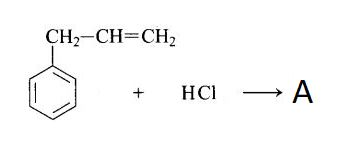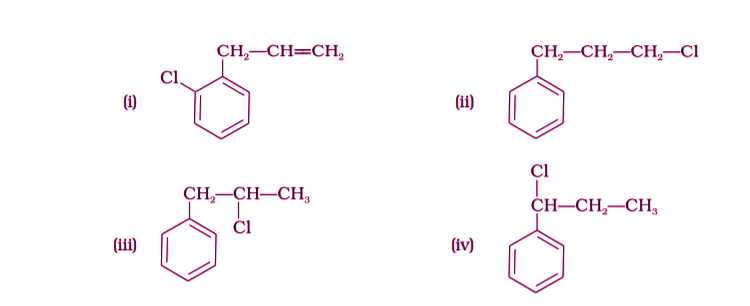Solution:

In the above reaction, the addition of HCl across the double bond takes place as per the Markovnikov rule. According to Markovnikov rule, the negative part of the adding molecule gets attached to that carbon atom which possesses a lesser number of hydrogen atoms.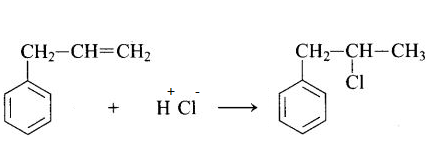16. A primary alkyl halide would prefer to undergo _____________.

(i) SN1 reaction

(ii) SN2 reaction

(iii) α–Elimination

(iv) Racemisation

Solution:

17. Which of the following alkyl halides will undergo SN1 reaction most readily?

(i) (CH3)3C—F

(ii) (CH3)3C—Cl

(iii) (CH3)3C—Br

(iv) (CH3)3C—I

Solution:

18. Which is the correct IUPAC name for?

(i) 1-Bromo-2-ethylpropane

(ii) 1-Bromo-2-ethyl-2-methylethane

(iii) 1-Bromo-2-methylbutane

(iv) 2-Methyl-1-bromobutane

Solution:

19. What should be the correct IUPAC name for diethylbromomethane?

(i) 1-Bromo-1,1-diethylmethane

(ii) 3-Bromopentane

(iii) 1-Bromo-1-ethylpropane

(iv) 1-Bromopentane

Solution:

20. The reaction of toluene with chlorine in the presence of iron and the absence

of light yields ____________.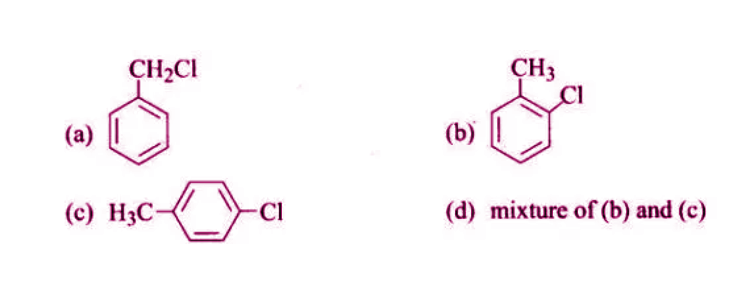Solution:

21. Chloromethane on treatment with an excess of ammonia yields mainly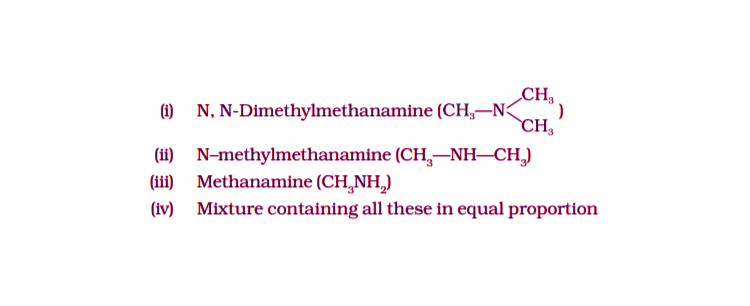Solution:

22. Molecules whose mirror image is non-superimposable over them are known

as chiral. Which of the following molecules is chiral?

(i) 2-Bromobutane

(ii) 1-Bromobutane

(iii) 2-Bromopropane

(iv) 2-Bromopropan-2-ol

Solution:

23. Reaction of C6H5CH2Br with aqueous sodium hydroxide follows ____________.

(i) SN1 mechanism

(ii) SN2 mechanism

(iii) Any of the above two depending upon the temperature of the reaction

(iv) Saytzeff rule

Solution:

24. Which of the carbon atoms present in the molecule given below are asymmetric?(i) a, b, c, d

(ii) b, c

(iii) a, d

(iv) a b, c

Solution:

25. Which of the following compounds will give racemic mixture on nucleophilic

substitution by OH– ion?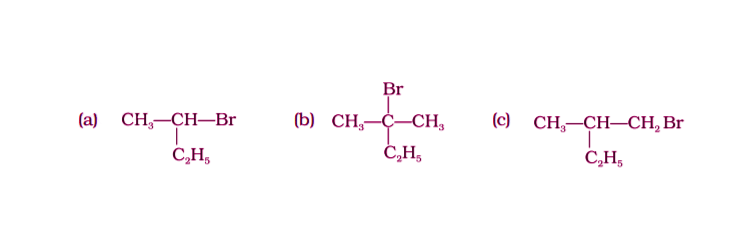(i) (a)

(ii) (a), (b), (c)

(iii) (b), (c)

(iv) (a), (c)

Solution:

Note: In the questions, 26 to 29 arrange the compounds in increasing order

of the rate of reaction towards nucleophilic substitution.

26.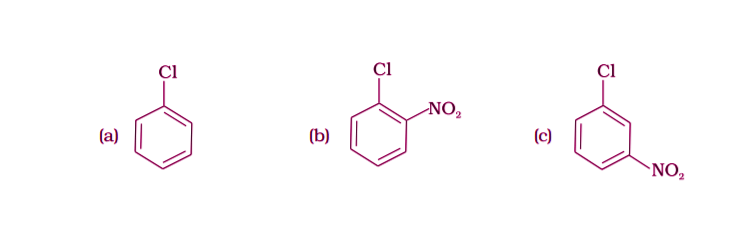(i) (a) < (b) < (c)

(ii) (c) < (b) < (a)

(iii) (a) < (c) < (b)

(iv) (c) < (a) < (b)

Solution:

27.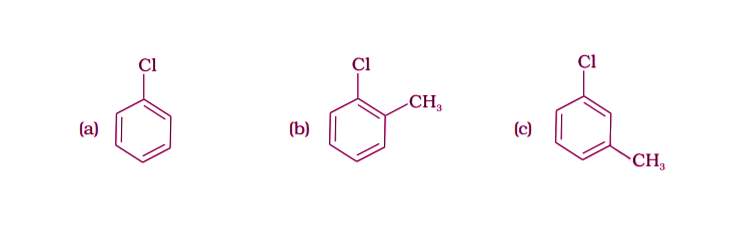(i) (a) < (b) < (c)

(ii) (a) < (c) < (b)

(iii) (c) < (b) < (a)

(iv) (b) < (c) < (a)

Solution:

28.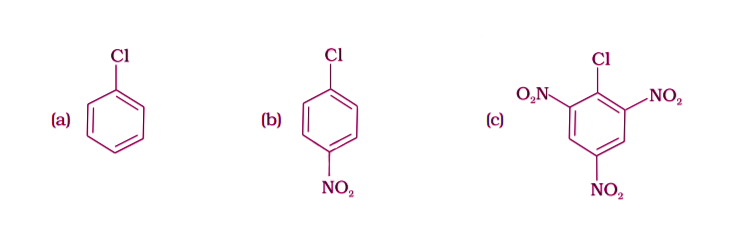(i) (c) < (b) < (a)

(ii) (b) < (c) < (a)

(iii) (a) < (c) < (b)

(iv) (a) < (b) < (c)

Solution:

29.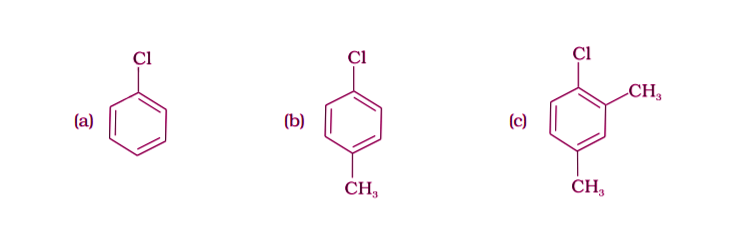(i) (a) < (b) < (c)

(ii) (b) < (a) < (c)

(iii) (c) < (b) < (a)

(iv) (a) < (c) < (b)

Solution:

30. Which is the correct increasing order of boiling points of the following

compounds?

1-Iodobutane, 1-Bromobutane, 1-Chlorobutane, Butane

(i) Butane < 1-Chlorobutane < 1-Bromobutane < 1-Iodobutane

(ii) 1-Iodobutane < 1-Bromobutane < 1-Chlorobutane < Butane

(iii) Butane < 1-Iodobutane < 1-Bromobutane < 1-Chlorobutane

(iv) Butane < 1-Chlorobutane < 1-Iodobutane < 1-BromobutaneSolution:

31. Which is the correct increasing order of boiling points of the following compounds?

1-Bromoethane, 1-Bromopropane, 1-Bromobutane, Bromobenzene

(i) Bromobenzene < 1-Bromobutane < 1-Bromopropane < 1-Bromoethane

(ii) Bromobenzene < 1-Bromoethane < 1-Bromopropane < 1-Bromobutane

(iii) 1-Bromopropane < 1-Bromobutane < 1-Bromoethane < Bromobenzene

(iv) 1-Bromoethane < 1-Bromopropane < 1-Bromobutane < Bromobenzene

Solution:

II. Multiple Choice Questions (Type-II)

Note: In the following questions two or more options may be correct.

Consider the following reaction and answer the questions no. 32–34.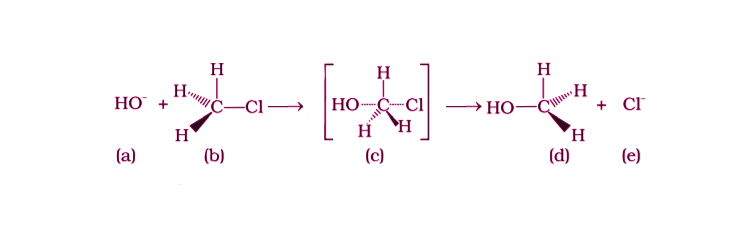32. Which of the statements are correct about the above reaction?

(i) (a) and (e) both are nucleophiles.

(ii) In (c) carbon atom is sp3 hybridised.

(iii) In (c) carbon atom is sp2 hybridised.

(iv) (a) and (e) both are electrophiles.

Solution:

Option (i) and (iii) are the answers.

(i) The given reaction follows the SN2 mechanism.

(ii) (b) and (d) have the opposite configuration.

(iii) (b) and (d) have the same configuration.

(iv) The given reaction follows the SN1 mechanism.

Solution:

Option (i) and (ii) are the answers.

34. Which of the following statements are correct about the reaction intermediate?

(i) Intermediate (c) is unstable because in this carbon is attached to 5 atoms.

(ii) Intermediate (c) is unstable because carbon atom is sp2

hybridised.

(iii) Intermediate (c) is stable because carbon atom is sp2

hybridised.

(iv) Intermediate (c) is less stable than the reactant (b).

Solution:

Option (i) and (iv) are the answers.

Answer Q. No. 35 and 36 based on the following reaction.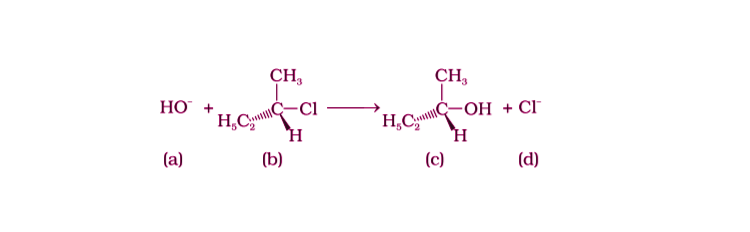35. Which of the following statements are correct about the mechanism of this reaction?

(i) A carbocation will be formed as an intermediate in the reaction.

(ii) OH–will attach the substrate (b) from one side and Cl- will leave it simultaneously from the other side.

(iii) An unstable intermediate will be formed in which OH– and Cl– will be attached by weak bonds.

(iv) The reaction proceeds through an SN1 mechanism.

Solution:

Option (i) and (iv) are the answers.

36. Which of the following statements are correct about the kinetics of this

reaction?

(i) The rate of reaction depends on the concentration of only (b).

(ii) The rate of reaction depends on the concentration of both (a) and (b).

(iii) Molecularity of reaction is one.

(iv) Molecularity of reaction is two.

Solution:

Option (i) and (iii) are the answers.

37. Haloalkanes contain halogen atom (s) attached to the sp3 hybridised carbon atom of an alkyl group. Identify haloalkane from the following compounds.

(i) 2-Bromopentane

(ii) Vinyl chloride (chloroethene)

(iii) 2-chloroacetophenone

(iv) Trichloromethane

Solution:

Option (i) and (iv) are the answers.

38. Ethylene chloride and ethylidene chloride are isomers. Identify the correct

statements.

(i) Both the compounds form the same product on treatment with alcoholic KOH.

(ii) Both the compounds form the same product on treatment with aq.NaOH.

(iii) Both the compounds form the same product on reduction.

(iv) Both the compounds are optically active.

Solution:

Option (i) and (iii) are the answers.

39. Which of the following compounds are gem-dihalides?

(i) Ethylidene chloride

(ii) Ethylene dichloride

(iii) Methylene chloride

(iv) Benzyl chloride

Solution:

Option (i) and (iii) are the answers.

40. Which of the following are secondary bromides?

(i) (CH3)2 CHBr

(ii) (CH3)3C CH2Br

(iii) CH3CH(Br)CH2CH3

(iv) (CH3)2CBrCH2CH3

Solution:

Option (i) and (iii) are the answers.

41. Which of the following compounds can be classified as aryl halides?

(i) p-ClC6H4CH2CH(CH3)2

(ii) p-CH3CHCl(C6H4)CH2CH3

(iii) o-BrH2C-C6H4CH(CH3)CH2CH3

(iv) C6H5-Cl

Solution:

Option (i) and (iv) are the answers.

42. Alkyl halides are prepared from alcohols by treating with

(i) HCl + ZnCl2

(ii) Red P + Br2

(iii) H2SO4+ KI

(iv) All the above

Solution:

Option (i) and (ii) are the answers

43. Alkyl fluorides are synthesised by heating an alkyl chloride/bromide in

presence of ____________ or ____________.

(i) Ca F2

(ii) CoF2

(iii) Hg2F2

(iv) NaF

Solution:

Option (ii) and (iii) are the answers.

44. Aryl chlorides and bromides can be easily prepared by electrophilic substitution of arenes with chlorine and bromine respectively in the presence of Lewis acid catalysts. But why does the preparation of aryl iodides requires the presence of an oxidising agent?

Solution:

Iodination of arenes is reversible due to the formation of HI. To move the reaction forward, an oxidizing agent like HNO3 or HIO4 oxidises HI, thus stabilizing the product.

45. Out of o-and p-bromobenzene which one has a higher melting point and why?

Solution:

p-bromobenzene has a higher melting point because the symmetry of p-dibromobenzene makes the molecule fit better in a crystal lattice. So that it requires a higher temperature to break the bonds between the molecules and thus has a higher melting point.

46. Which of the compounds will react faster in SN1 reaction with the –OH ion?

CH3— CH2— Cl or C6H5— CH2— Cl

Solution:

C6H5— CH2— Cl will react faster in an SN1 reaction with the OH- ion. This happens due to the stability of the carbocation in the compound. C6H5 group is already stable due to resonance, and the CH2 attached will gain that stability, thus forming a stable C6H5CH2+ carbocation after the cleavage in the first step of the SN1 reaction.

47. Why iodoform has appreciable antiseptic property?

Solution:

Due to the liberation of free iodine iodoform has an appreciable antiseptic property.

48. Haloarenes are less reactive than haloalkanes and haloalkenes. Explain.

Solution:

This is because of the resonance stabilization of the aryl ring. If we take an example of C6H5-Cl, there will be a conjugation of chlorine electrons with the electrons in the ring. This resonance makes C-Cl bond to get partial double bond character and makes less reactive to nucleophilic substitution.

49. Discuss the role of Lewis acids in the preparation of aryl bromides and chlorides in the dark.

Solution:

Aryl bromides and chlorides can be prepared from arenes by electrophilic substitution. This reaction is carried out by treating the arene with chlorine or bromine in the presence of iron (III) chloride in the absence of light. Iron (III) chloride, is a Lewis acid, which generates the electrophile required to take the reaction forward. FeCl3 forms a coordination compound with Cl2, making the complex Cl+[FeCl4-].

50. Which of the following compounds (a) and (b) will not react with a mixture of

NaBr and H2SO4. Explain why?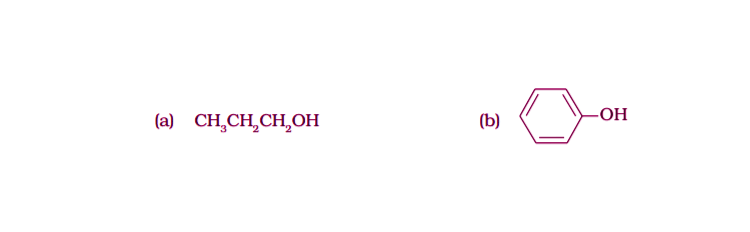Solution:

A mixture of NaBr and H2SO4 gives Br2 gas as a product. Molecule (b) will not react with Br2 gas because of the stable molecule that is formed due to resonance stabilization.

51. Which of the products will be a major product in the reaction given below?

Explain.

CH3CH=CH2 + HI → CH3CH2CH2I + CH3CHICH30

(A) (B)

Solution:

The molecule (B) will be the major product in the reaction. This addition reaction is carried out by following Markovnikoff’s rule, wherein a double bond, the hydrogen from the hydrogen halide is added to the carbon atom with the most hydrogen atoms attached to it,

52. Why is the solubility of haloalkanes in water very low?

Solution:

Haloalkanes are very slightly soluble in water because, to dissolve a haloalkane in water, energy is required to overcome the attractions between the haloalkane molecules and also to break the hydrogen bonds between water molecules.

53. Draw other resonance structures related to the following structure and find out whether the functional group present in the molecule is ortho, para directing or meta directing.Solution: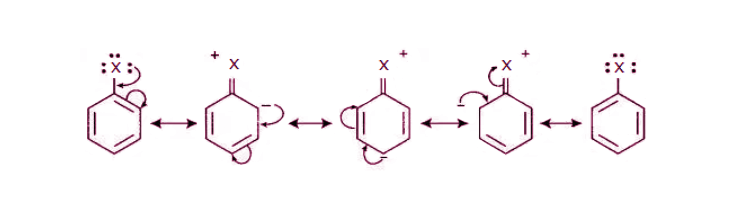The functional group present in these molecules are ortho-para directing as electron density is more at ortho and para positions.

54. Classify the following compounds as primary, secondary and tertiary halides.

(i) 1-Bromobut-2-ene

(ii) 4-Bromopent-2-ene

(iii) 2-Bromo-2-methylpropane

Solution:

(i) 1-Bromobut-2-ene is a primary halide

(ii) In 4-Bromopent-2-ene, the bromine is attached to the secondary carbon atom, hence this a secondary halide.

(iii) In 2-Bromo-2-methylpropane, the bromine is attached to the tertiary carbon atom, making it a tertiary halide.

55. Compound ‘A’ with molecular formula C4H9Br is treated with aq. KOH solution.

The rate of this reaction depends upon the concentration of the compound ‘A’

only. When another optically active isomer ‘B’ of this compound was treated

with aq. KOH solution, the rate of reaction was found to be dependent on

the concentration of compound and KOH both.

(i) Write down the structural formula of both compounds ‘A’ and ‘B’.

(ii) Out of these two compounds, which one will be converted to the product

with an inverted configuration.

Solution: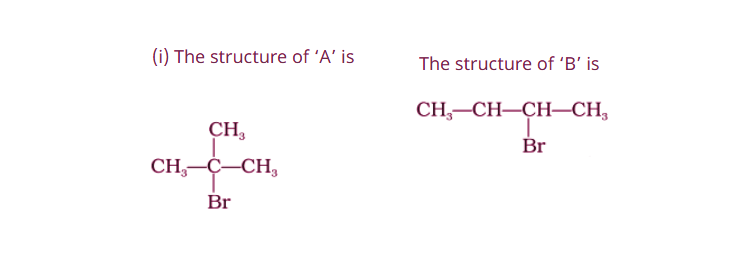(ii) Compound ‘B’ will undergo inversion of configuration and give an inverted product because it undergoes SN2 reaction.

### The Class 12 NCERT Exemplar Problems on Haloalkanes and Haloarenes

Studying the NCERT Class 12 Chemistry Exemplar for Chapter 10 PDF aids you in preparing notes on the chapter. This page has answers to exemplar questions in the textbook, question papers and sample papers. MCQs, short answer questions, match the following and other exercises help you in preparing for the graduate entrance exams, such as NEET, AIPMT, JEE, AIEE, and state entrance exams.
Key concepts to remember

1. Classification – Based on the Number of Halogen Atoms, Compounds Containing sp3 C—X Bond (X= F, Cl, Br, I), Compounds Containing sp2 C—X Bond
2. Nomenclature – Structure, Common Name and IUPAC Name
3. Nature of C-X Bond- Carbon-Halogen (C—X) Bond Lengths, Bond Enthalpies and Dipole Moments
4. Methods of Preparation – From Alcohols and Hydrocarbons
5. Physical Properties- Melting Points, Boiling Points, Melting Points and Solubility
6. Chemical Reactions – Nucleophilic Substitution, Elimination Reactions and Reaction with Metals
7. Polyhalogen Compounds

Download The NCERT Class 12 Chemistry Exemplar for Chapter 10 Haloalkanes and Haloarenes from the links below.

#### Why Opt for BYJU’S

BYJU’S provides Chapter-wise NCERT Solutions and study materials for all subjects of all classes. BYJU’S also provides assistance to clear the doubts of CBSE students. Our subject experts will reach out to students to clarify their doubts. If you have any doubts regarding study materials, you can also contact our support team at any time.